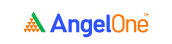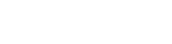# Mean, median and modeIn this chapter, we’ll travel back in time a little and revisit three basic statistical concepts. Mean, median, and mode. Rings a bell? These three metrics are quite central to understanding the correlation between two stocks. Without further ado, let’s get to discussing these statistical tools. We’ll check out the mean, median, and mode formula and check out some examples on how to find the mean, median and mode.

### Pair trading statistics: An elementary example

Meet 12-year old Raghu. He’s in the sixth grade, and he’s not the most studious guy in class. He likes to catch up on his extracurriculars and he loves playing outdoors. But Raghu is not unintelligent, either. He pays attention in class and he gets his homework done on time. All in all, he’s your typical average student.

Let’s look at the marks he obtained over the last ten tests.

 Test number Marks obtained (out of 100) 1 83 2 75 3 91 4 64 5 52 6 83 7 97 8 68 9 49 10 76 Total 738

### Calculating the mean

The mean is also commonly known as the average. Simply put, it represents the average value in a group of data points. It is calculated as the sum of all the data points, divided by the total number of data points.

Here’s the formula.

 Arithmetic mean = (Sum of all observations) ÷ (Total number of observations)

In the case of Raghu and his marks we saw earlier, the arithmetic mean can be calculated as follows.

• We’re looking at 10 tests.
• The total of all the marks Raghu obtained over those 10 tests is 738.

So, the arithmetic mean for this set of observations is:

= 738 ÷ 10

= 73.8

### Calculating the median: Scenario 1

The median is another fundamental statistical tool. In layman terms, it represents the middle value in a set of distributions. To figure out what the median is, the given data points first need to be arranged in ascending order. Then, here’s how you calculate the median.

• If there is an odd number of observations, the median is the observation that features in the middle of the arrangement.
• If there is an even number of observations, the median is the average of the two middle data points.

To understand this better, let’s calculate the median for Raghu’s marks across the 10 tests.

Step 1: Arranging the observations in order

 49 52 64 68 75 76 83 83 91 97

Step 2: Identifying the middle value(s)

Here, since we have 10 observations, there are two middle data points - the fifth and the sixth numbers. You can see they’ve been highlighted for easier understanding.

• So, 75 and 76 are the two middle data points.
• The average of these two is the median.

Step 3: Calculating the median

In this case, the median is calculated as the average of 75 and 76, which is:

= (75 + 76) ÷ 2

= 75.5

So, in this scenario, Raghu’s median mark is 75.5.

### Calculating the median: Scenario 2

Let’s rejig the data points we have originally taken and assume that Raghu has taken 11 tests (instead of 10) during the year. Here are the revised marks for the 11 tests.

 Test number Marks obtained (out of 100) 1 83 2 75 3 91 4 64 5 52 6 83 7 97 8 68 9 49 10 76 11 63 Total 801

Here’s how the median calculation goes for this set of observations.

Step 1: Arranging the observations in order

 49 52 63 64 68 75 76 83 83 91 97

Step 2: Identifying the middle value(s)

Here, since we have 11 observations, there is just one middle data point - the sixth number. Here too, it has been highlighted for easier understanding.

Step 3: Calculating the median

In this case, the median is the sixth data point, which is 75.

So, Raghu’s median mark in this scenario is 75.

### Calculating the mode

The mode is perhaps the easiest statistical tool. It requires no calculations or complicated math. If you’re like most people, the mode may also have been your favorite of the three tools we’re discussing in this chapter.

So, let’s see what this statistical metric represents. It is simply the observation that occurs the maximum number of times.

In the case of Raghu’s marks, you’ll see that off the 10 (or the 11) tests he’s taken, he has obtained a score of 83 in two tests. That makes 83 the mode for this distribution.

### Correlation calculation: A quick primer

Now, you may have been wondering why we’ve been discussing the mean, median and mode formula. As with everything else in the earlier chapters, these concepts and discussions all have one key intent - to figure out how to establish the correlation between two stocks. As the dust settles, things will get clearer in the upcoming chapters.

Before that, however, let’s take a quick look at some ways to calculate the correlation between two stocks. If you have a basic level of proficiency in Microsoft Excel, you’ll find it easy enough.

Now, the actual calculator - that’s easy. You just need to type out a predetermined function in your spreadsheet. The real work lies in getting the data points for the correlation calculator ready. You can use any of the following observations to calculate the correlation between two stocks:

• The closing prices of the stocks
• The daily change in their closing prices
• The daily return they deliver

Let’s look at these three cases in detail.

### 1. Calculating the correlation based on the closing prices

Let’s take up the two stocks we’ve become familiar with over the previous chapter in this module - TCS and Infosys. The screenshot below shows you the closing prices of the two stocks over a one-month period.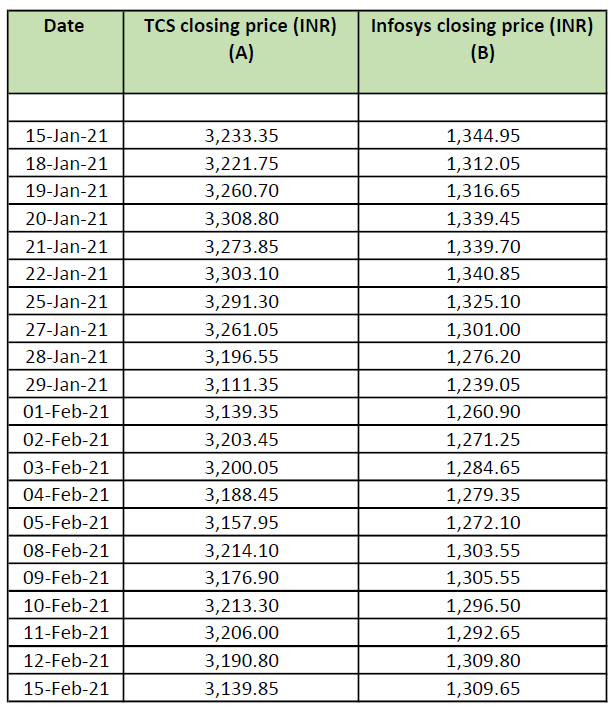And here’s how you use the correlation function to calculate the correlation between TCS and Infosys shares based on their closing prices.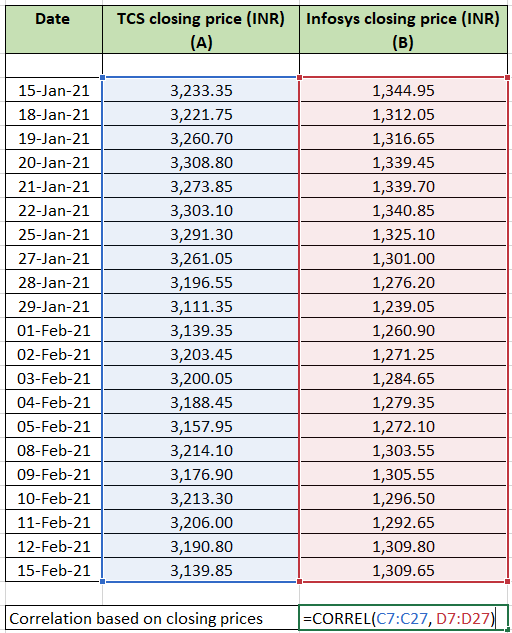When you use the correlation function for the given data points, the correlation between TCS and Infosys turns out to be 0.79, which is quite strong.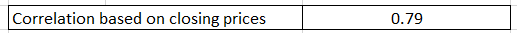### 2. Calculating the correlation based on the daily change in closing prices

The daily change in closing prices is calculated as the difference between the previous day’s closing price and the present day’s closing price. The screenshot below shows you the daily changes in the closing prices of the two stocks over the same one-month period.And once again, we’ll use the correlation function to calculate the correlation between TCS and Infosys shares based on the daily changes in their closing prices.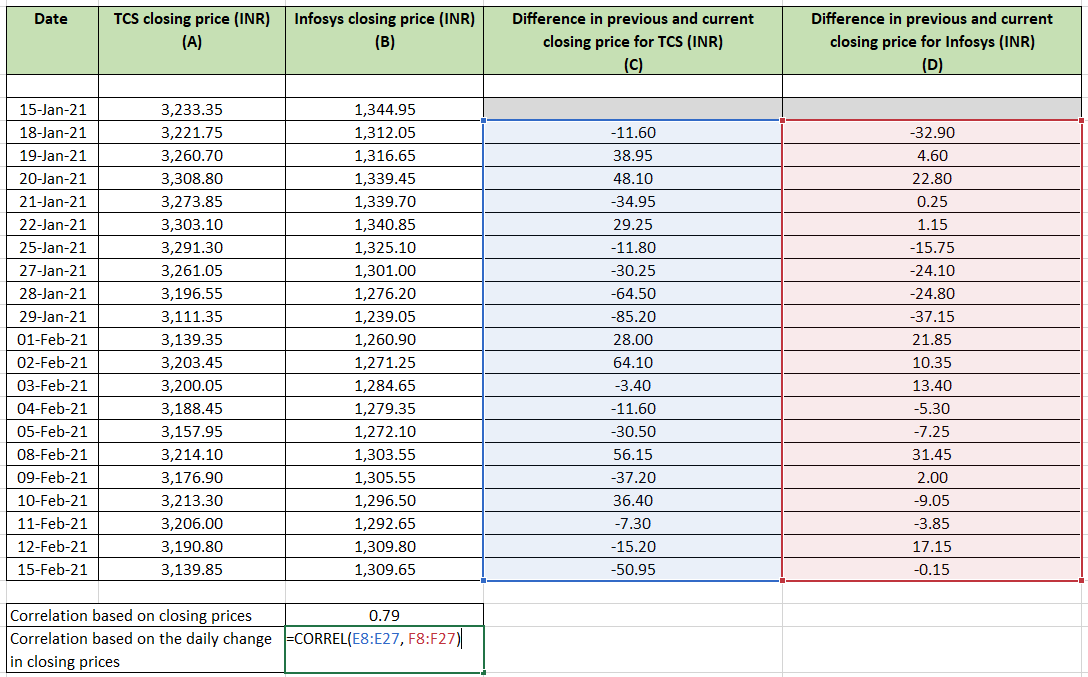On using the correlation function for the given data points, the correlation between TCS and Infosys turns out to be 0.67, which is, at best, average.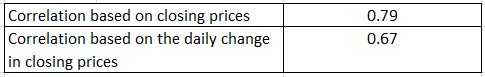### 3. Calculating the correlation based on the daily return delivered by the stocks

The daily return is a measure of the gain (or the loss) that the daily price movements of a stock bring in. It is expressed as a percentage, and it’s calculated using the formula given below.

 Daily return:  (Today’s closing price - Previous day’s closing price) ÷ Previous day’s closing price

The screenshot below shows you the daily return delivered by the TCS and Infosys stocks over the period we’ve considered.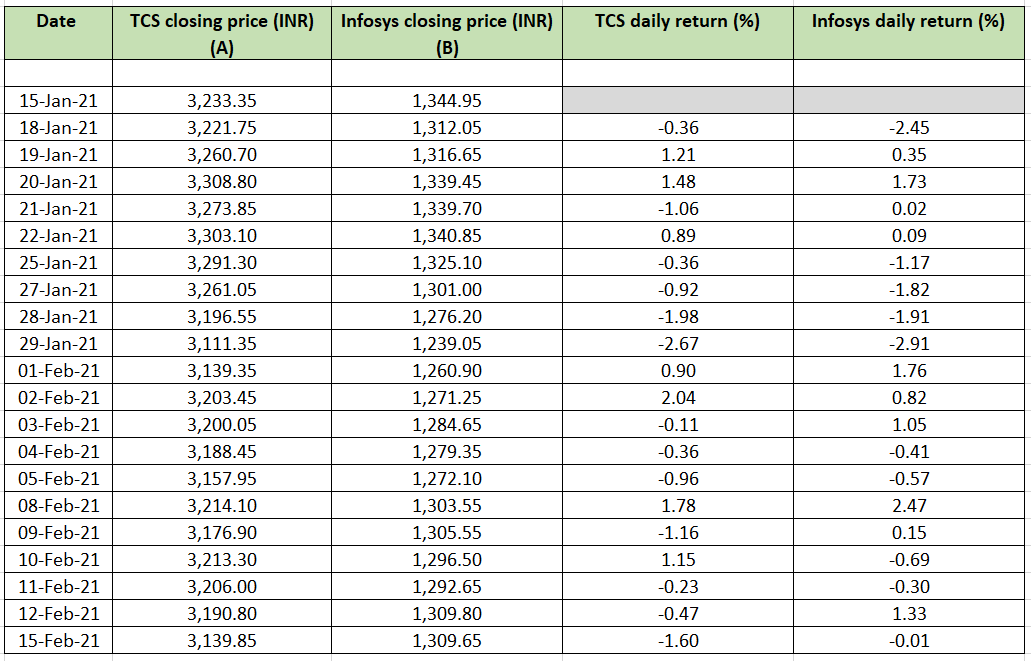And how, let’s use the correlation function to calculate the correlation between TCS and Infosys shares based on their daily returns.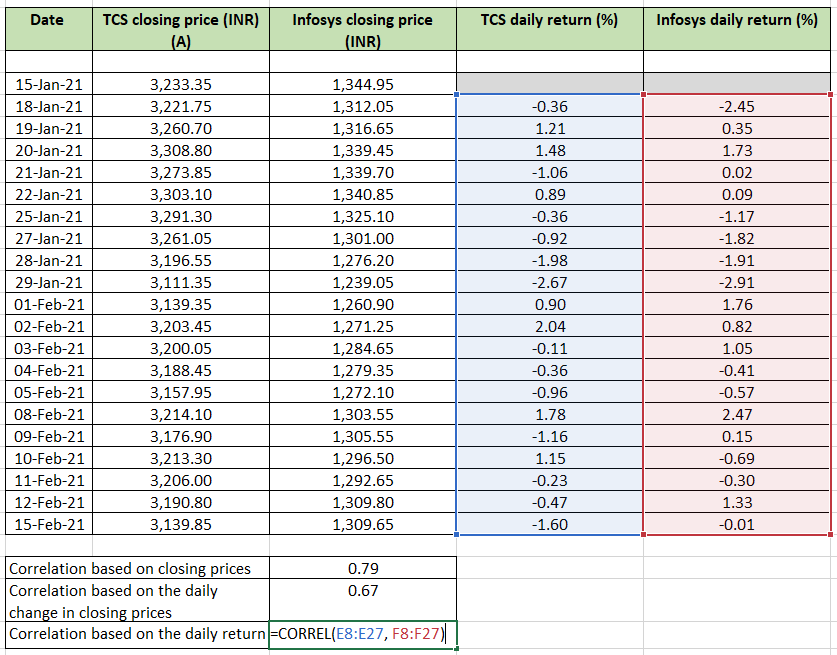So, by using the correlation function for the given data points, we see that the correlation between TCS and Infosys turns out to be around 0.67 again, which is quite average.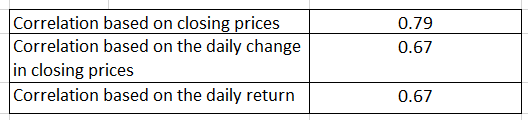#### Wrapping up

This should give you a fair idea of how the calculation for correlation is generally done. Now, as we head on to the next chapter, we’ll build up from the mean, median and mode formula and explore more statistical tools to understand pair trading better. Stay tuned!

#### A quick recap

• The mean represents the average value in a group of data points. It is calculated as the sum of all the data points, divided by the total number of data points.
• The median represents the middle value in a set of distributions. To figure out what the median is, the given data points first need to be arranged in ascending order.
• If there is an odd number of observations, the median is the observation that features in the middle of the arrangement.
• If there is an even number of observations, the median is the average of the two middle data points.
• The mode is the observation that occurs the maximum number of times in a set of data points.
• To calculate the correlation between two stocks, you can use the closing prices of the stocks, the daily change in their closing prices or the daily return they deliver.Take the quiz for this chapter & mark it complete.

How would you rate this chapter?

Get Information Mindfulness!

Catch-up With Market

News in 60 Seconds.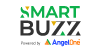The perfect starter to begin and stay tuned with your learning journey anytime and anywhere.